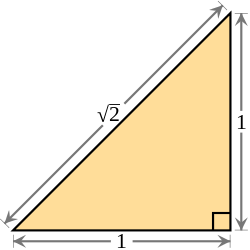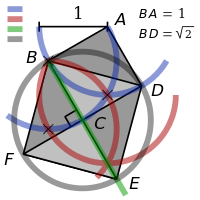# Constructible number

For numbers "constructible" in the sense of set theory, see Constructible universe.The square root of 2 is equal to the length of the hypotenuse of a right triangle with legs of length 1 and is therefore a constructible numberFor example, the square root of 2 is constructible: from the length unit, we can construct a line segment of length 2 with straightedge and compass.

A point in the Euclidean plane is a constructible point if, given a fixed coordinate system (or a fixed line segment of unit length), the point can be constructed with unruled straightedge and compass. A complex number is a constructible number if its corresponding point in the Euclidean plane is constructible from the usual x- and y-coordinate axes.

It can then be shown that a real number r is constructible if and only if, given a line segment of unit length, a line segment of length |r| can be constructed with compass and straightedge. It can also be shown that a complex number is constructible if and only if its real and imaginary parts are constructible.

In terms of algebra, a number is constructible if and only if it can be written using the four basic arithmetic operations and the extraction of square roots but of no higher-order roots. The set of constructible numbers can be completely characterized in the language of field theory: the constructible numbers form the quadratic closure of the rational numbers: the smallest field extension that is closed under square root and complex conjugation. This has the effect of transforming geometric questions about compass and straightedge constructions into algebra. This transformation leads to the solutions of many famous mathematical problems, which defied centuries of attack.

## Geometric definitions

The geometric definition of a constructible point is as follows. First, for any two distinct points P and Q in the plane, let L(P, Q ) denote the unique line through P and Q, and let C (P, Q ) denote the unique circle with center P, passing through Q. (Note that the order of P and Q matters for the circle.) By convention, L(P, P ) = C (P, P ) = {P }. Then a point Z is constructible from E, F, G and H if either

1. Z is in the intersection of L(E, F ) and L(G, H ), where L(E, F ) ≠ L(G, H );
2. Z is in the intersection of C (E, F ) and C (G, H ), where C (E, F ) ≠ C (G, H );
3. Z is in the intersection of L(E, F ) and C (G, H ).

Since the order of E, F, G, and H in the above definition is irrelevant, the four letters may be permuted in any way. Put simply, Z is constructible from E, F, G and H if it lies in the intersection of any two distinct lines, or of any two distinct circles, or of a line and a circle, where these lines and/or circles can be determined by E, F, G, and H, in the above sense.

Now, let A and A be any two distinct fixed points in the plane. A point Z is constructible if either

1. Z = A;
2. Z = A;
3. there exist points P0, ..., Pn, with Z = Pn, such that for all j ≥ 1, Pj + 1 is constructible from points in the set {P0, ..., Pj} where P0 = A and P1 = A.

Put simply, Z is constructible if it is either A or A, or if it is obtainable from a finite sequence of points starting with A and A, where each new point is constructible from previous points in the sequence.

For example, the center point of A and A is defined as follows. The circles C (A, A) and C (A, A) intersect in two distinct points; these points determine a unique line, and the center is defined to be the intersection of this line with L(A, A).

## Transformation into algebra

All rational numbers are constructible, and all constructible numbers are algebraic numbers. Also, if a and b are constructible numbers with b ≠ 0, then a ± b , a×b, ab, and a and are constructible. In particular, the set K of all constructible complex numbers forms a field, a subfield of the field of algebraic numbers. A complex number is constructible if and only if the real and imaginary parts are both constructible.

Furthermore, K is closed under square roots and complex conjugation. These facts can be used to characterize the field of constructible numbers, because, in essence, the equations defining lines and circles are no worse than quadratic. The characterization is the following: a complex number is constructible if and only if it lies in a field at the top of a finite tower of quadratic extensions, starting with the rational field Q. More precisely, z is constructible if and only if there exists a tower of fieldswhere z is in Kn and for all 0 ≤ j < n, [Kj + 1:Kj ] = 2.

## Trigonometric numbers

Main article: Trigonometric number

Trigonometric numbers are irrational cosines or sines of angles that are rational multiples of π. Such a number is constructible if and only if the denominator of the fully reduced multiple is a power of 2 or the product of a power of 2 with the product of one or more distinct Fermat primes. Thus, for example, cos(π/15) Is constructible because 15 is the product of the two Fermat primes: 3 and 5.

See here a list of trigonometric numbers expressed in terms of square roots.

## Impossible constructions

The algebraic characterization of constructible numbers provides an important necessary condition for constructibility: if z is constructible, then it is algebraic, and its minimal irreducible polynomial has degree a power of 2, or equivalently, the field extension Q(z)/Q has dimension a power of 2. One should note that it is true, (but not obvious to show) that the converse is false this is not a sufficient condition for constructibility. However, this defect can be remedied by considering the normal closure of Q(z)/Q.

The non-constructibility of certain numbers proves the impossibility of certain problems attempted by the philosophers of ancient Greece. In the following chart, each row represents a specific ancient construction problem. The left column gives the name of the problem. The second column gives an equivalent algebraic formulation of the problem. In other words, the solution to the problem is affirmative if and only if each number in the given set of numbers is constructible. Finally, the last column provides the simplest known counterexample. In other words, the number in the last column is an element of the set in the same row, but is not constructible.

Construction problem Associated set of numbers Counterexample
Doubling the cubeis not constructible, because its minimal polynomial has degree 3 over Q
Trisecting the angleis not constructible, becausehas minimal polynomial of degree 3 over Q
Squaring the circleis not constructible, because it is not algebraic over Q
Constructing all regular polygonsis not constructible, because 7 is not a Fermat prime, nor is 7 the product ofand one or more Fermat primes